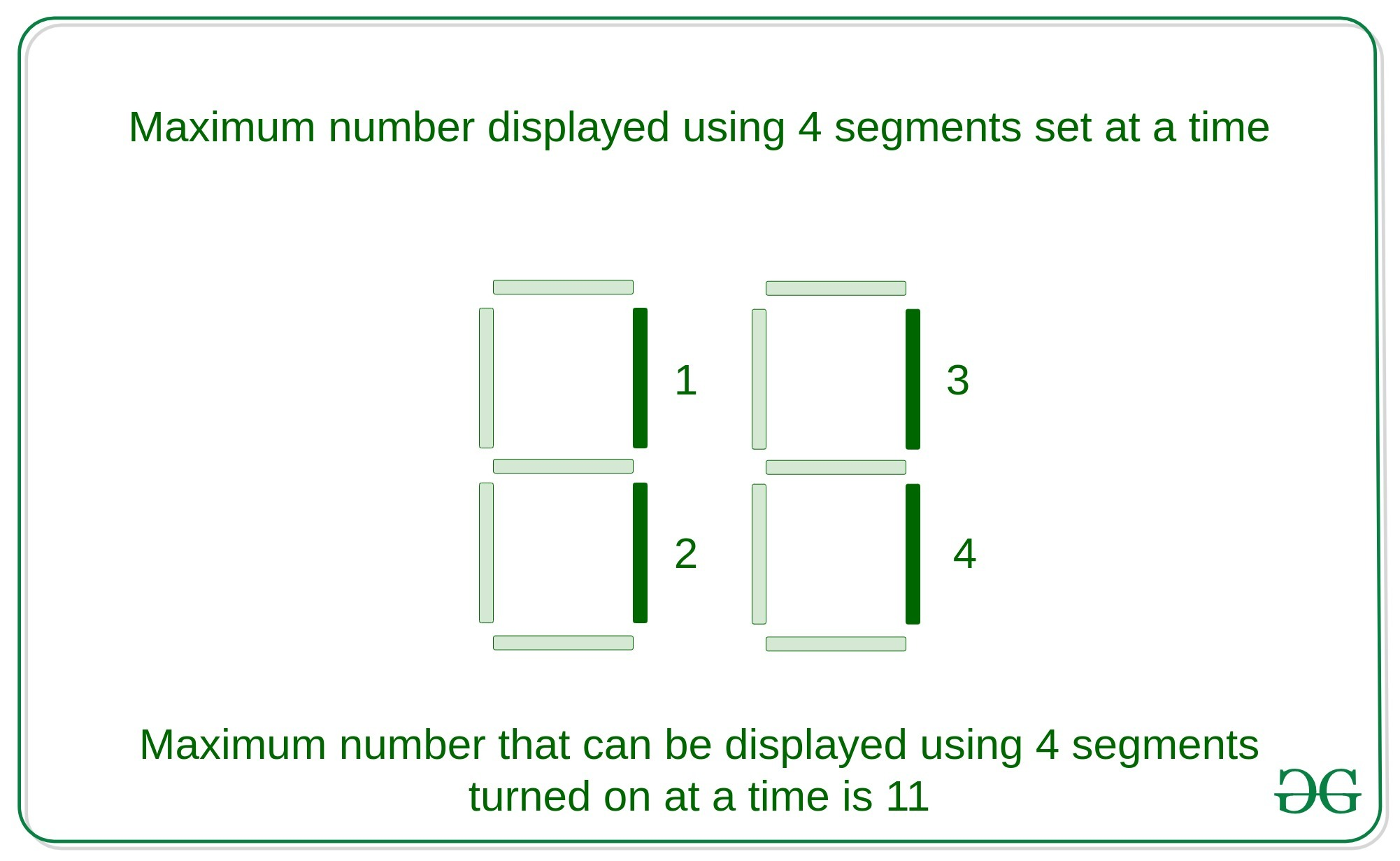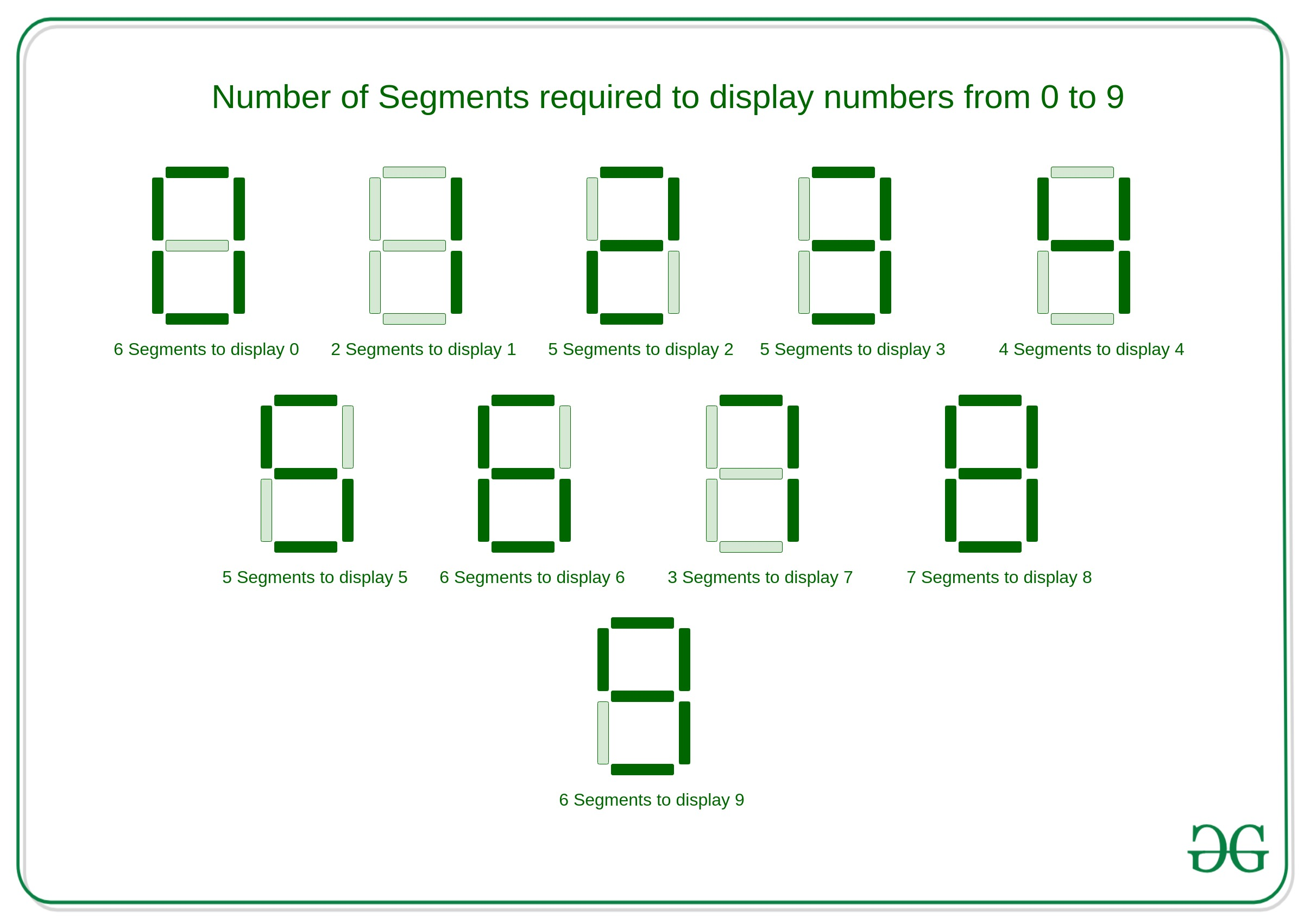Open in App
Not now

# Maximum number on 7-segment display using N segments : Recursive

• Last Updated : 16 Dec, 2022

Given an integer N, the task is to find the largest number that can be shown with the help of N segments using any number of 7 segment displays.
Examples:

Input: N = 4
Output: 11
Explanation:
Largest number that can be displayed with the help of 4 segments using 2 seven segment displays turned is 11.Input: N = 7
Output: 711
Explanation:
Largest number that can be displayed by turning on seven segments is 711 with the help of 3 segments display set.

Approach:
The key observation in seven segment display is to turn on any number from 0 to 9 takes certain amounts of segments, which is described below:If the problem is observed carefully, then the number N can be of two types that is even or odd and each of them should be solved separately as follows:

• For Even: As in the above image, There are 6 numbers that can be displayed using even number of segments which is

```0 - 6
1 - 2
2 - 5
4 - 4
6 - 6
9 - 6```
• As it is observed number 1 uses the minimum count of segments to display a digit. Then, even the number of segments can be displayed using 1 with 2 counts of segments in each digit.

• For Odd: As in the above image, there are 5 numbers that can be displayed using an odd number of segments which is

```3 - 5
5 - 5
7 - 3
8 - 7```
• As it is observed number 7 uses the minimum number of odd segments to display a digit. Then an odd number of segments can be displayed using 7 with 3 counts of segments in each digit.

Algorithm:

• If the given number N is 0 or 1, then any number cannot be displayed with this much of bits.
• If the given number N is odd then the most significant digit will be 7 and the rest of the digits can be displayed with the help of the (N – 3) segments because to display 7 it takes 3 segments.
• If given number N is even then the most significant digit will be 1 and the rest of the digits can be displayed with the help of the (N – 2) segments because to display 1, it takes 2 segments only.
• The number N is processed digit by digit recursively.

Explanation with Example:
Given number N be – 11

Then, the largest number will be 71111.
Below is the implementation of the above approach:

## C++

 `// C++ implementation to find the``// maximum number that can be``// using the N segments in``// N segments display` `#include ``using` `namespace` `std;` `// Function to find the maximum``// number that can be displayed``// using the N segments``void` `segments(``int` `n)``{``    ``// Condition to check base case``    ``if` `(n == 1 || n == 0) {``        ``return``;``    ``}``    ``// Condition to check if the``    ``// number is even``    ``if` `(n % 2 == 0) {``        ``cout << ``"1"``;``        ``segments(n - 2);``    ``}``    ` `    ``// Condition to check if the``    ``// number is odd``    ``else` `if` `(n % 2 == 1) {` `        ``cout << ``"7"``;``        ``segments(n - 3);``    ``}``}` `// Driver Code``int` `main()``{``    ``int` `n;``    ``n = 11;``    ``segments(n);``    ``return` `0;``}`

## Java

 `// Java implementation to find the``// maximum number that can be``// using the N segments in``// N segments display``import` `java.io.*;``class` `GFG {``    ` `    ``// Function to find the maximum``    ``// number that can be displayed``    ``// using the N segments``    ``static` `void` `segments(``int` `n)``    ``{``        ``// Condition to check base case``        ``if` `(n == ``1` `|| n == ``0``) {``            ``return``;``        ``}``        ``// Condition to check if the``        ``// number is even``        ``if` `(n % ``2` `== ``0``) {``            ``System.out.print(``"1"``);``            ``segments(n - ``2``);``        ``}``        ` `        ``// Condition to check if the``        ``// number is odd``        ``else` `if` `(n % ``2` `== ``1``) {``    ` `            ``System.out.print(``"7"``);``            ``segments(n - ``3``);``        ``}``    ``}``    ` `    ``// Driver Code``    ``public` `static` `void` `main (String[] args)``    ``{``        ``int` `n;``        ``n = ``11``;``        ``segments(n);``    ``}``}` `// This code is contributed by AnkitRai01`

## Python3

 `# Python3 implementation to find the``# maximum number that can be``# using the N segments in``# N segments display` `# Function to find the maximum``# number that can be displayed``# using the N segments``def` `segments(n) :` `    ``# Condition to check base case``    ``if` `(n ``=``=` `1` `or` `n ``=``=` `0``) :``        ``return``;``    ` `    ``# Condition to check if the``    ``# number is even``    ``if` `(n ``%` `2` `=``=` `0``) :``        ``print``(``"1"``,end``=``"");``        ``segments(n ``-` `2``);``    ` `    ``# Condition to check if the``    ``# number is odd``    ``elif` `(n ``%` `2` `=``=` `1``) :` `        ``print``(``"7"``,end``=``"");``        ``segments(n ``-` `3``);` `# Driver Code``if` `__name__ ``=``=` `"__main__"` `:` `    ``n ``=` `11``;``    ``segments(n);` `# This code is contributed by AnkitRai01`

## C#

 `// C# implementation to find the``// maximum number that can be``// using the N segments in``// N segments display``using` `System;` `class` `GFG {``    ` `    ``// Function to find the maximum``    ``// number that can be displayed``    ``// using the N segments``    ``static` `void` `segments(``int` `n)``    ``{``        ``// Condition to check base case``        ``if` `(n == 1 || n == 0) {``            ``return``;``        ``}``        ``// Condition to check if the``        ``// number is even``        ``if` `(n % 2 == 0) {``            ``Console.Write(``"1"``);``            ``segments(n - 2);``        ``}``        ` `        ``// Condition to check if the``        ``// number is odd``        ``else` `if` `(n % 2 == 1) {``    ` `            ``Console.Write(``"7"``);``            ``segments(n - 3);``        ``}``    ``}``    ` `    ``// Driver Code``    ``public` `static` `void` `Main()``    ``{``        ``int` `n;``        ``n = 11;``        ``segments(n);``    ``}``}` `// This code is contributed by AnkitRai01`

## Javascript

 ``

Output:

`71111`

Performance Analysis:

• Time Complexity: As in the above approach, there is recursive call which takes O(N) time in worst case, Hence the Time Complexity will be O(N).
• Auxiliary Space Complexity: As in the above approach, taking consideration of the stack space used in recursive call then the auxiliary space complexity will be O(N)

My Personal Notes arrow_drop_up